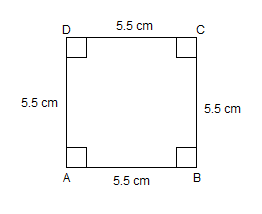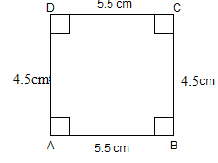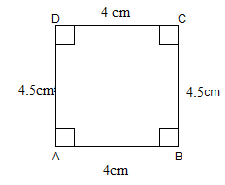If construct a square, each of whose sides measures 5.5 cm , then it should be like,

# If construct a square, each of whose sides measures 5.5 cm , then it should be like,

1. A2. B3. C4. D
None of these

Fill Out the Form for Expert Academic Guidance!l

+91

Live ClassesBooksTest SeriesSelf Learning

Verify OTP Code (required)

### Solution:

The properties of basic quadrilaterals such as parallelogram, square, rectangle, rhombus, etc. should be remembered as these are common shapes whose constructions are required. In the above question, you need to remember all the properties of squares to construct it. It doesn't say we have to use a compass, so you can use a protractor to construct 90 degrees. However, you need to know how to construct angles of 30°, 45°, 60°, 90°, 120° because sometimes there is no point in using a protractor to construct these angles.
So, if construct a square, each of whose sides measures 5.5 cm , then it should be likeis right.
So option (1) is the correct answer.

## Related content

 Area of Square Area of Isosceles Triangle Pythagoras Theorem Triangle Formula Perimeter of Triangle Formula Area Formulae Volume of Cone Formula Matrices and Determinants_mathematics Critical Points Solved Examples Type of relations_mathematics+91

Live ClassesBooksTest SeriesSelf Learning

Verify OTP Code (required)